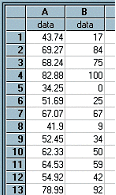﻿ Percentile(column)

# Percentile(column)

Top  Previous  Next
 Calculates the percentile (or percent rank) of each data point in column.  The percentile represents the percentage of scores in the data set that are less than or equal to the data point.   Column B in the example contains the percent ranks of scores in column A.